# RD Sharma Solutions for Class 11 Chapter 13 - Complex Numbers Exercise 13.3

In this exercise, we shall deal with problems based on the square roots of a complex number. Students can make use of the RD Sharma Class 11 Solutions while solving exercise wise problems. The solutions are designed by subject matter experts based on the student’s abilities where any student can understand the possible ways of obtaining answers easily. The PDF of RD Sharma Solutions for Class 11 Maths Chapter 13 Exercise 13.3 is available here. Students who aim to clear the exams with good marks can download the PDF from the given links.

## Download the pdf of RD Sharma Solutions for Class 11 Maths Exercise 13.3 Chapter 13 – Complex Numbers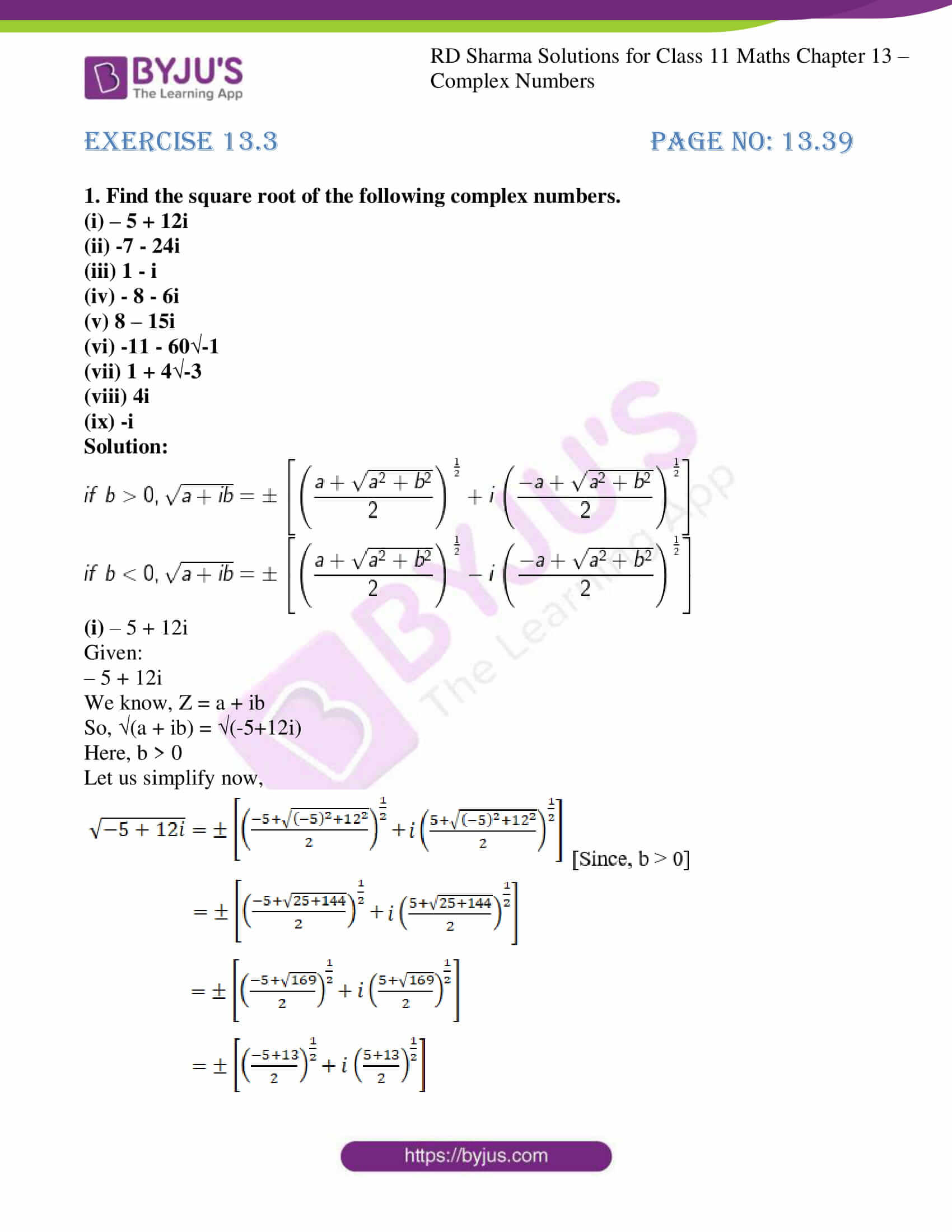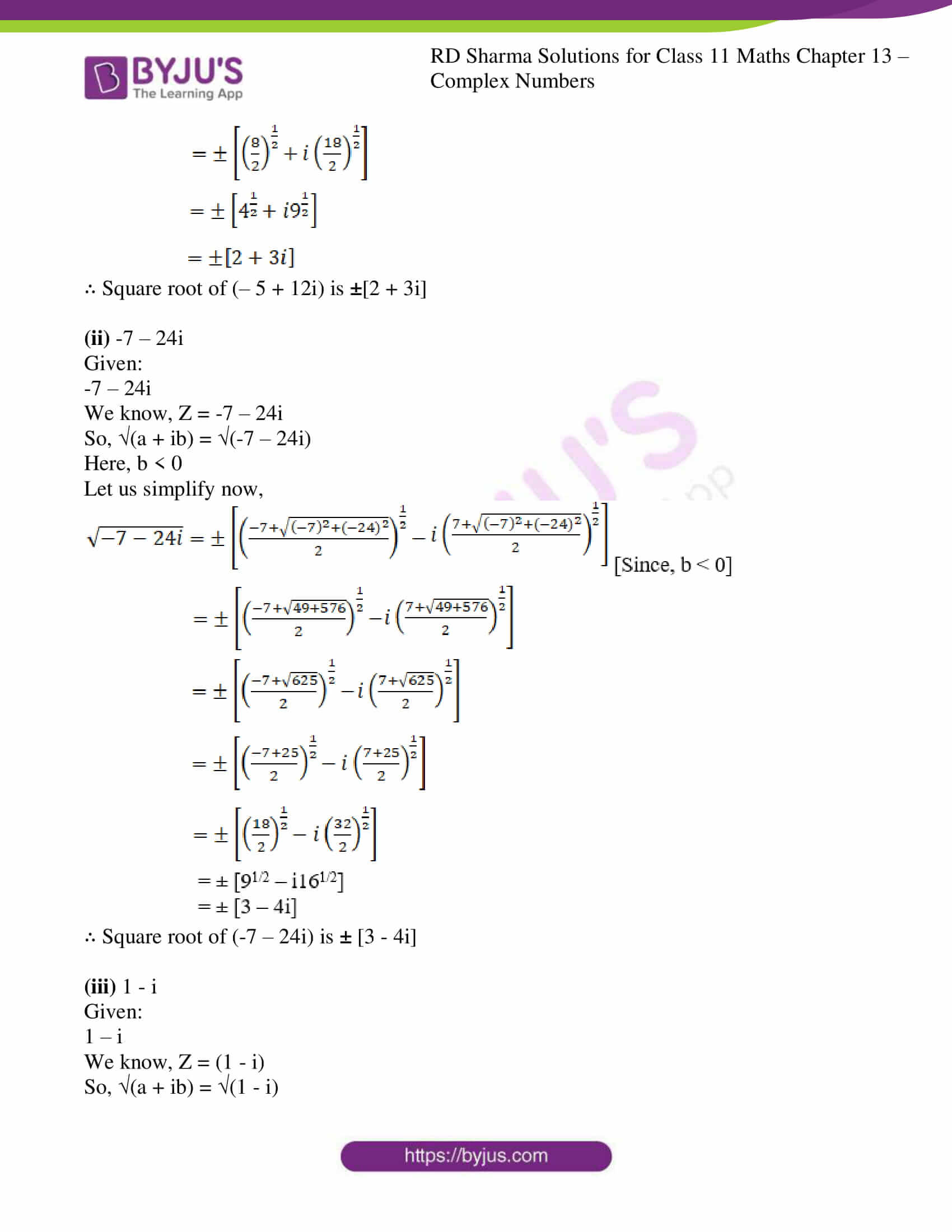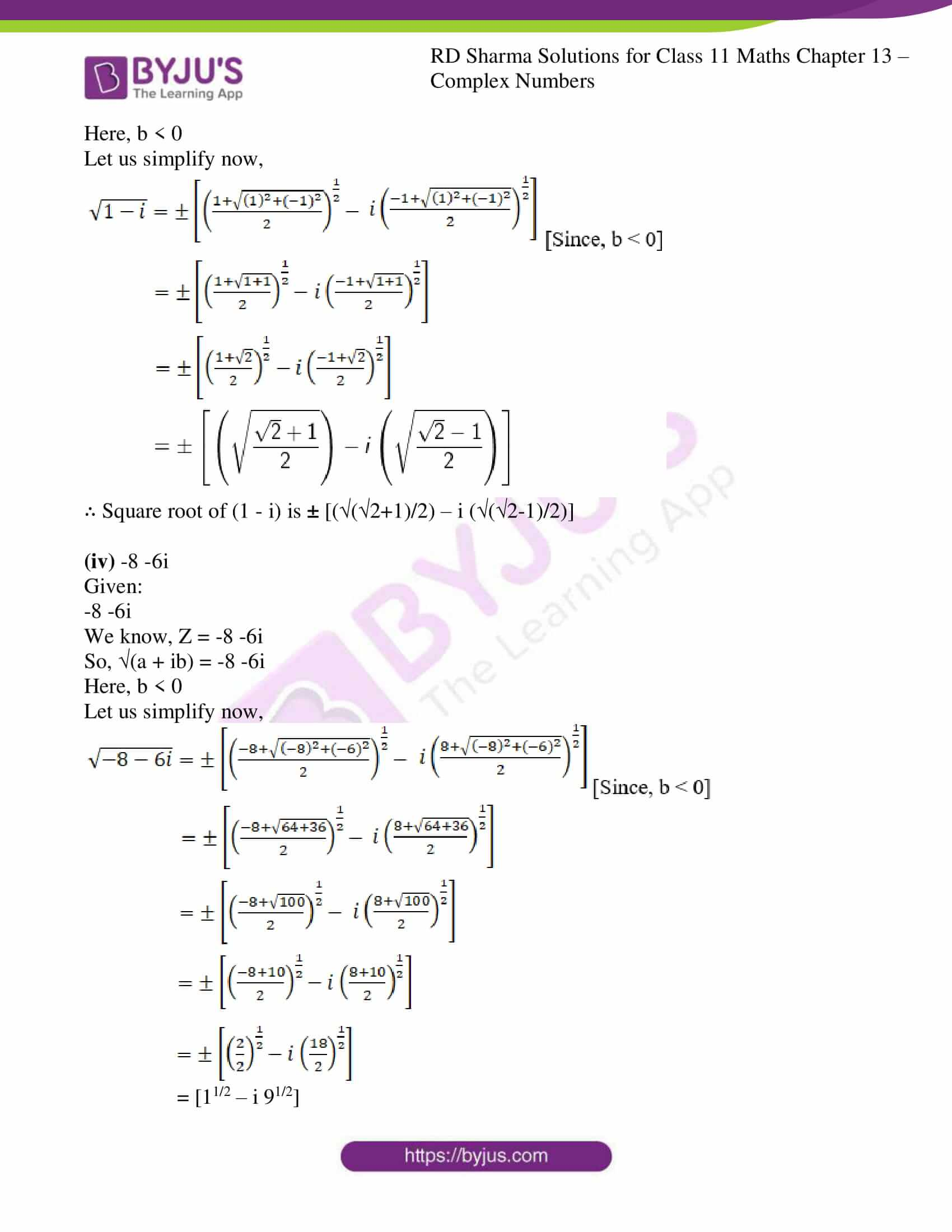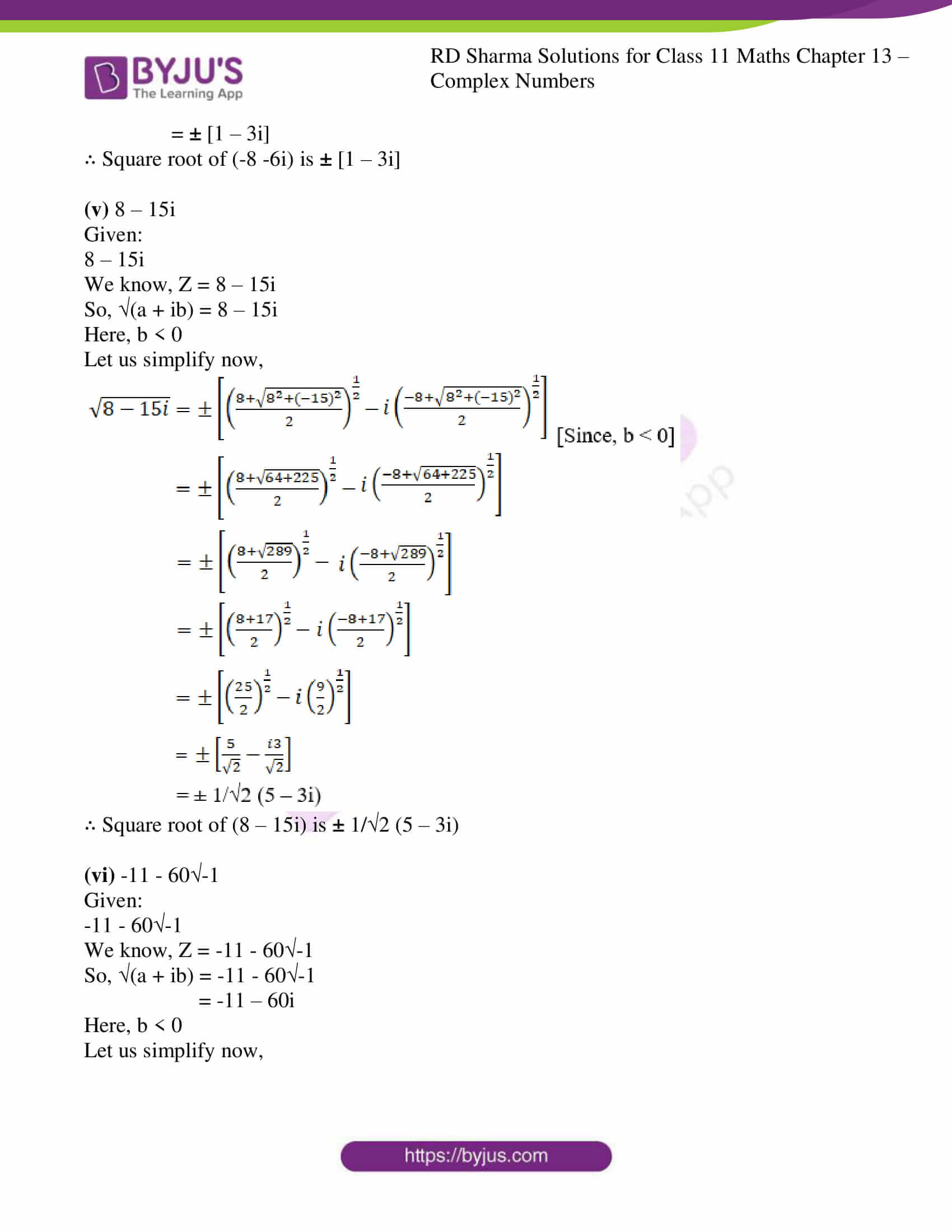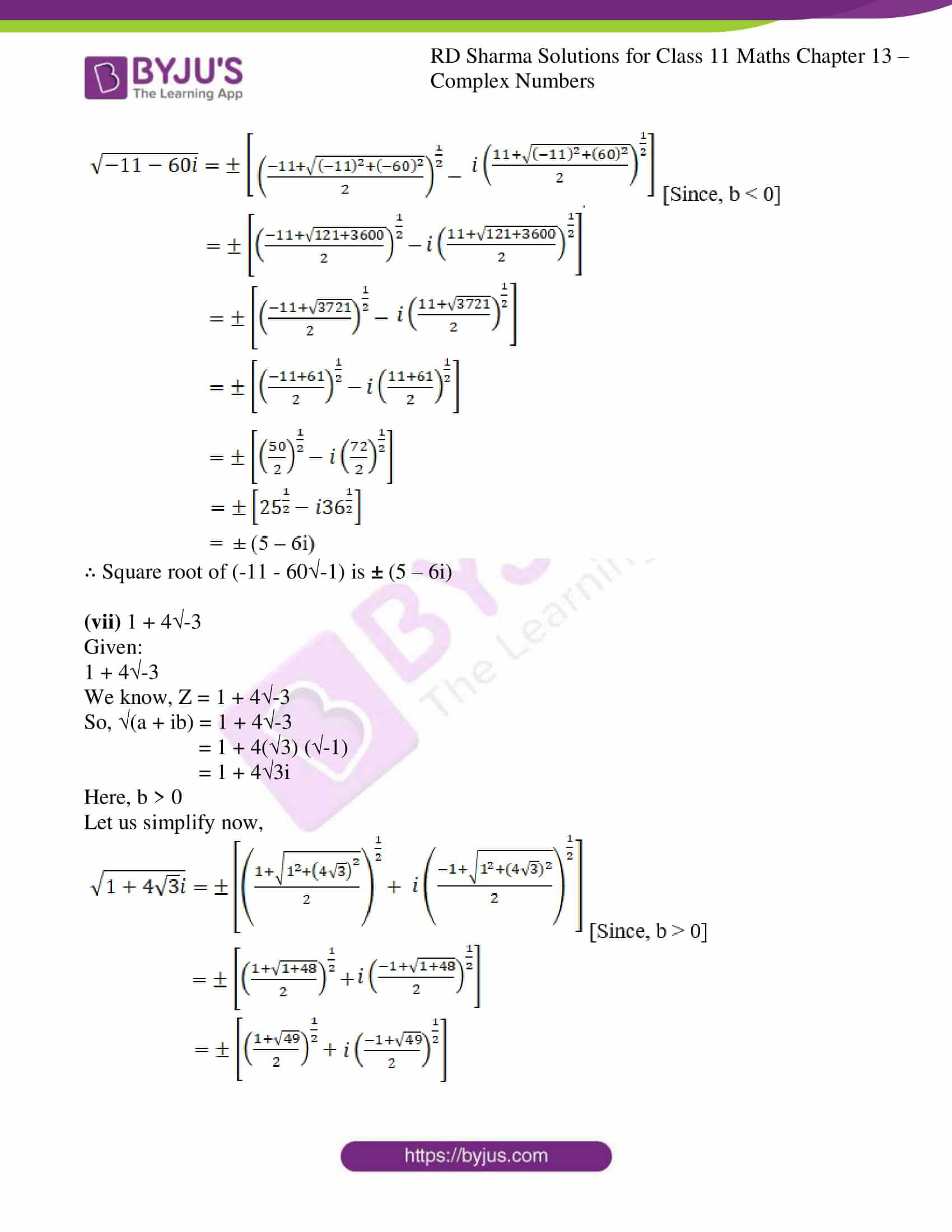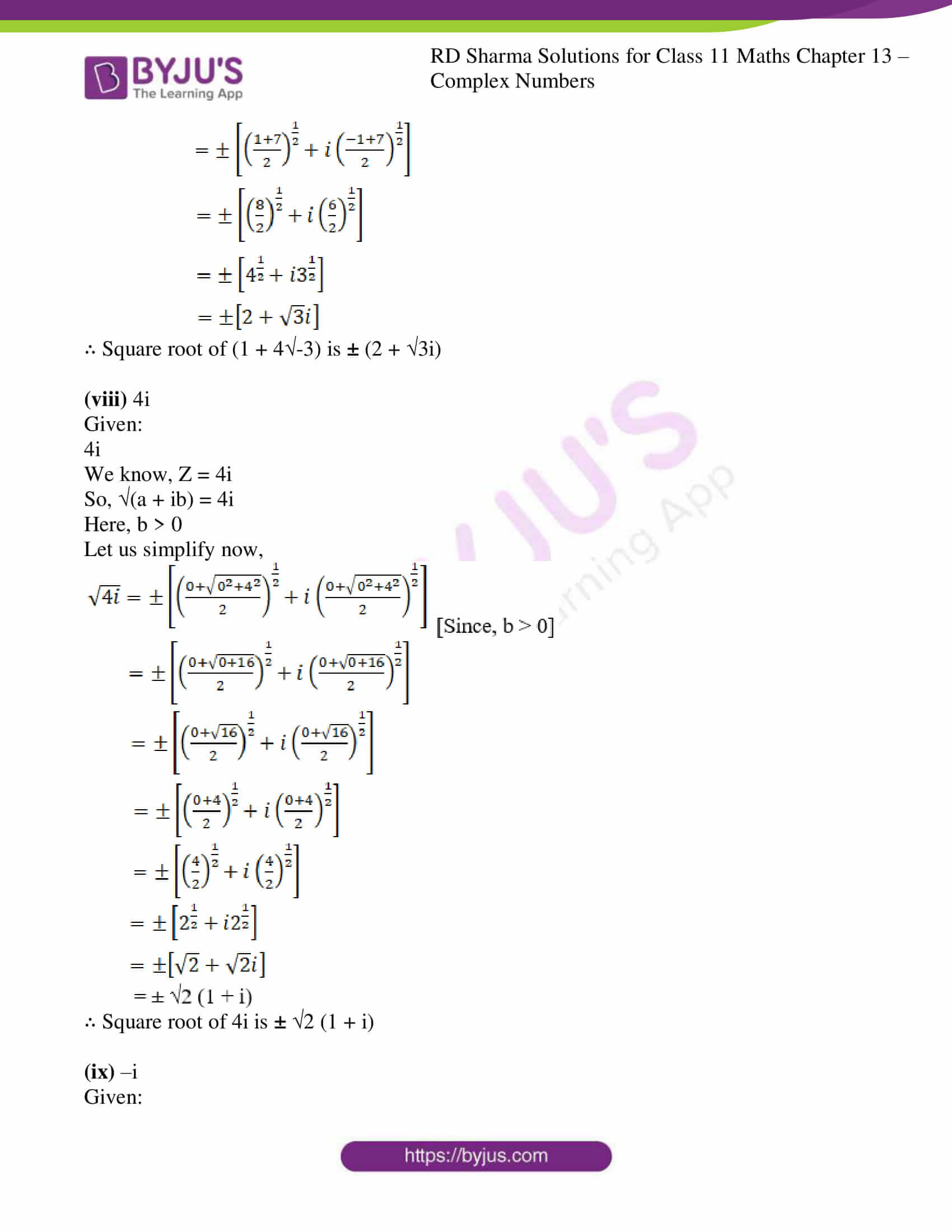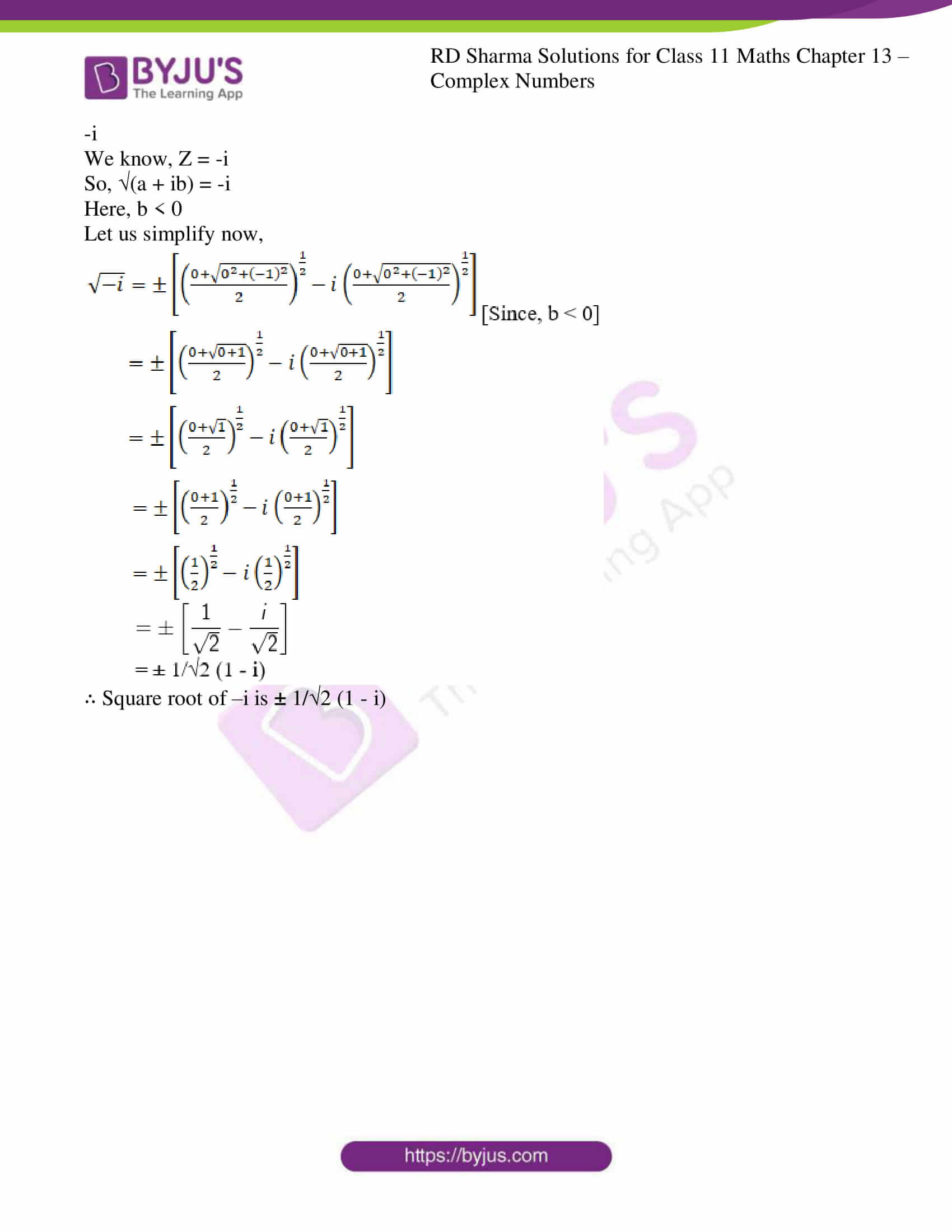### Access answers to RD Sharma Solutions for Class 11 Maths Exercise 13.3 Chapter 13 – Complex Numbers

1. Find the square root of the following complex numbers.

(i) – 5 + 12i

(ii) -7 – 24i

(iii) 1 – i

(iv) – 8 – 6i

(v) 8 – 15i

(vi) -11 – 60√-1

(vii) 1 + 4√-3

(viii) 4i

(ix) -i

Solution: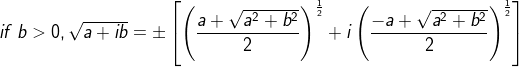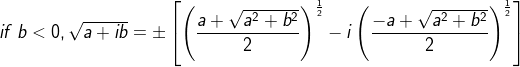(i) – 5 + 12i

Given:

– 5 + 12i

We know, Z = a + ib

So, (a + ib) = (-5+12i)

Here, b > 0

Let us simplify now,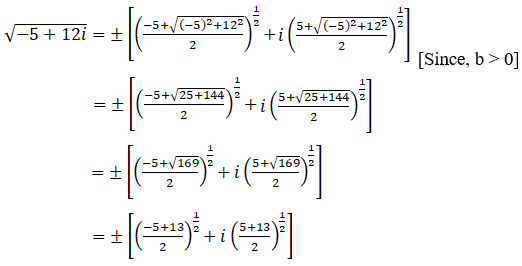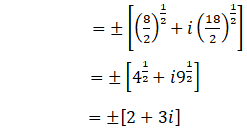∴ Square root of (– 5 + 12i) is ±[2 + 3i]

(ii) -7 – 24i

Given:

-7 – 24i

We know, Z = -7 – 24i

So, (a + ib) = √(-7 – 24i)

Here, b < 0

Let us simplify now,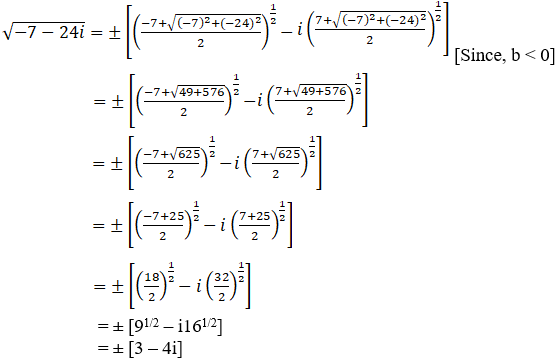∴ Square root of (-7 – 24i) is ± [3 – 4i]

(iii) 1 – i

Given:

1 – i

We know, Z = (1 – i)

So, (a + ib) = (1 – i)

Here, b < 0

Let us simplify now,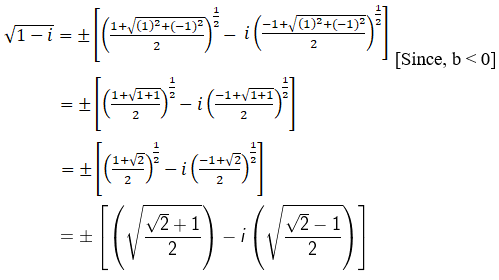∴ Square root of (1 – i) is ± [((2+1)/2) – i ((2-1)/2)]

(iv) -8 -6i

Given:

-8 -6i

We know, Z = -8 -6i

So, (a + ib) = -8 -6i

Here, b < 0

Let us simplify now,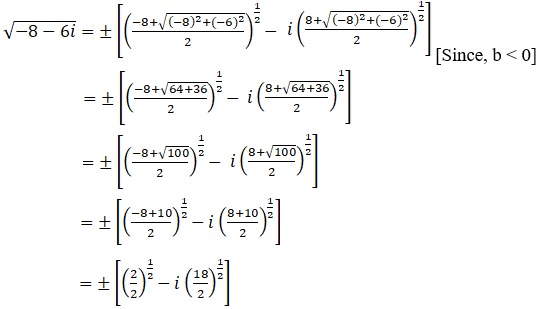= [11/2 – i 91/2]

= ± [1 – 3i]

∴ Square root of (-8 -6i) is ± [1 – 3i]

(v) 8 – 15i

Given:

8 – 15i

We know, Z = 8 – 15i

So, (a + ib) = 8 – 15i

Here, b < 0

Let us simplify now,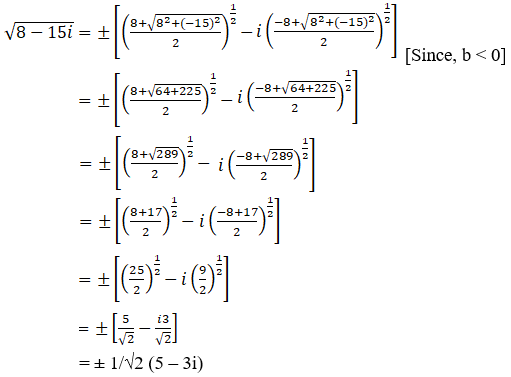∴ Square root of (8 – 15i) is ± 1/2 (5 – 3i)

(vi) -11 – 60-1

Given:

-11 – 60-1

We know, Z = -11 – 60-1

So, (a + ib) = -11 – 60-1

= -11 – 60i

Here, b < 0

Let us simplify now,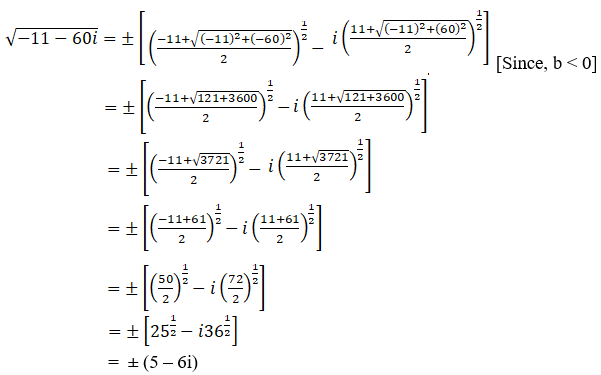∴ Square root of (-11 – 60-1) is ± (5 – 6i)

(vii) 1 + 4-3

Given:

1 + 4-3

We know, Z = 1 + 4-3

So, (a + ib) = 1 + 4-3

= 1 + 4(3) (-1)

= 1 + 43i

Here, b > 0

Let us simplify now,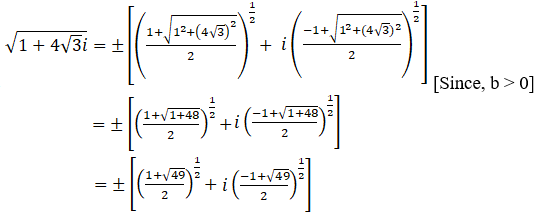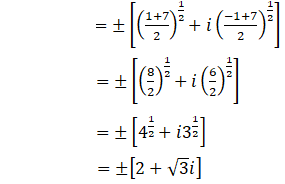∴ Square root of (1 + 4-3) is ± (2 + 3i)

(viii) 4i

Given:

4i

We know, Z = 4i

So, (a + ib) = 4i

Here, b > 0

Let us simplify now,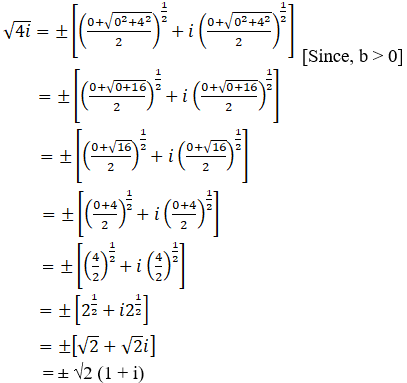∴ Square root of 4i is ± 2 (1 + i)

(ix) –i

Given:

-i

We know, Z = -i

So, (a + ib) = -i

Here, b < 0

Let us simplify now,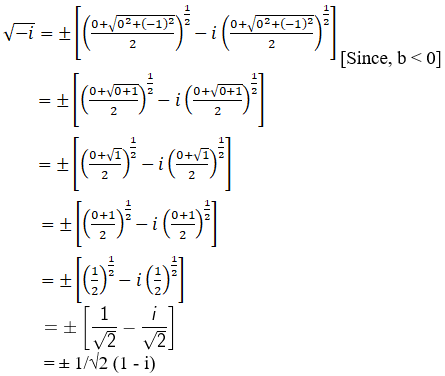∴ Square root of –i is ± 1/2 (1 – i)